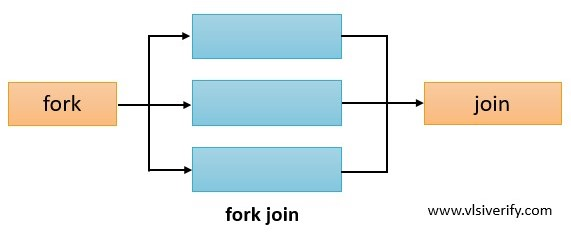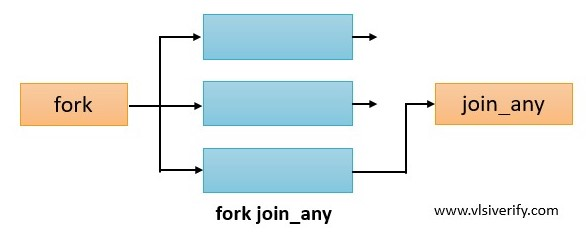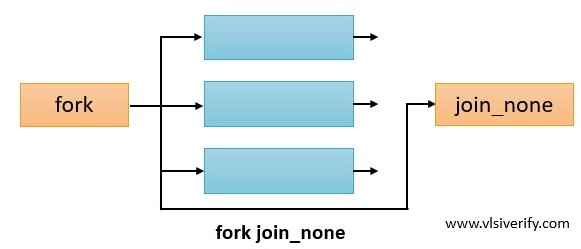Tutorials

The process or thread is a common concept of an operating system. In an operating system (OS), a process is a program (a piece of code) that is under execution.

Similarly in SystemVerilog, process or thread is also a piece of code that executes independently. The process or thread is enclosed in begin..end. If begin..end is not used, each statement acts as a separate process.

SystemVerilog provides three different ways to start/control processes.

## SystemVerilog fork join

In fork-join, all processes start simultaneously, and join will wait for all processes to be completed.### Example of fork join

In the below example, there are three processes A, B, and C. All started at the same time.

Execution time for process A: 10ns

Execution time for process B: 15ns

Execution time for process C: 20ns

Fork-join will be completed when all three process execution is completed.

``````module fork_join_example;

initial begin
fork
begin // process A
\$display("Process A started at time = %0t", \$time);
#10;
\$display("Process A completed at time = %0t", \$time);
end
begin // process B
\$display("Process B started at time = %0t", \$time);
#15;
\$display("Process B completed at time = %0t", \$time);
end
begin // process C
\$display("Process C started at time = %0t", \$time);
#20;
\$display("Process C completed at time = %0t", \$time);
end
join
\$display("fork-join completed at time = %0t", \$time);
end
endmodule``````

Output:

``````Process A started at time = 0
Process B started at time = 0
Process C started at time = 0
Process A completed at time = 10
Process B completed at time = 15
Process C completed at time = 20
fork-join completed at time = 20``````

## SystemVerilog fork join_any

In fork-join_any, all processes start simultaneously and join_any will wait for any one process to be completed.### Example of fork join_any

In the below example, there are three processes A, B, and C. All started at the same time.

Execution time for process A: 10ns

Execution time for process B: 15ns

Execution time for process C: 20ns

Fork-join_any will be completed when any one of the three process execution is completed.

``````module fork_join_any_example;

initial begin
fork
begin // process A
\$display("Process A started at time = %0t", \$time);
#10;
\$display("Process A completed at time = %0t", \$time);
end
begin // process B
\$display("Process B started at time = %0t", \$time);
#15;
\$display("Process B completed at time = %0t", \$time);
end
begin // process C
\$display("Process C started at time = %0t", \$time);
#20;
\$display("Process C completed at time = %0t", \$time);
end
join_any
\$display("fork-join_any completed at time = %0t", \$time);
end
endmodule``````

Output:

``````Process A started at time = 0
Process B started at time = 0
Process C started at time = 0
Process A completed at time = 10
fork-join_any completed at time = 10
Process B completed at time = 15
Process C completed at time = 20``````

## SystemVerilog fork join_none

In fork-join_none, all processes start simultaneously and join_none will not wait for any process to be completed.

So, we can say that fork-join and fork-join_any is blocked due to process execution time, whereas fork-join_none is not blocked due to any process.### Example of fork join_none

In the below example, there are three processes A, B, and C. All started at the same time.

Execution time for process A: 10ns

Execution time for process B: 15ns

Execution time for process C: 20ns

Fork-join_none will be completed without waiting for any process completion.

``````module fork_join_none_example;
initial begin
fork
begin // process A
\$display("Process A started at time = %0t", \$time);
#10;
\$display("Process A completed at time = %0t", \$time);
end
begin // process B
\$display("Process B started at time = %0t", \$time);
#15;
\$display("Process B completed at time = %0t", \$time);
end
begin // process C
\$display("Process C started at time = %0t", \$time);
#20;
\$display("Process C completed at time = %0t", \$time);
end
join_none
\$display("fork-join_none completed at time = %0t", \$time);
end
endmodule``````

Output:

``````fork-join_none completed at time = 0
Process A started at time = 0
Process B started at time = 0
Process C started at time = 0
Process A completed at time = 10
Process B completed at time = 15
Process C completed at time = 20``````

## disable fork

The ‘disable fork’ statement terminates all outstanding or active processes.

### Without disable fork

In the below example, fork-join_any is used without a wait fork statement. So, once process A is finished, immediately join_any is unblocked without waiting for process B and C to be completed and further statements are executed.

``````module fork_join_any_example;
initial begin
fork
begin // process A
\$display("Process A started at time = %0t", \$time);
#10;
\$display("Process A completed at time = %0t", \$time);
end
begin // process B
\$display("Process B started at time = %0t", \$time);
#15;
\$display("Process B completed at time = %0t", \$time);
end
begin // process C
\$display("Process C started at time = %0t", \$time);
#20;
\$display("Process C completed at time = %0t", \$time);
end
join_any
\$display("fork-join_any completed at time = %0t", \$time);
end
endmodule``````

Output:

``````Process A started at time = 0
Process B started at time = 0
Process C started at time = 0
Process A completed at time = 10
fork-join_any completed at time = 10
Process B completed at time = 15
Process C completed at time = 20``````

### With disable fork

After the addition of the ‘disable fork’ statement, processes B and C will get terminated immediately.

``````module disable_fork_example;
initial begin
fork
begin // process A
\$display("Process A started at time = %0t", \$time);
#10;
\$display("Process A completed at time = %0t", \$time);
end
begin // process B
\$display("Process B started at time = %0t", \$time);
#15;
\$display("Process B completed at time = %0t", \$time);
end
begin // process C
\$display("Process C started at time = %0t", \$time);
#20;
\$display("Process C completed at time = %0t", \$time);
end
join_any
disable fork;
\$display("fork-join_any completed at time = %0t", \$time);
end
endmodule``````

Output:

``````Process A started at time = 0
Process B started at time = 0
Process C started at time = 0
Process A completed at time = 10
fork-join_any completed at time = 10``````

## wait fork

The ‘wait fork’ statement is used to wait for all forked processes to be completed.

### Without wait fork

In the below example, fork-join_any is used without a wait fork statement. So, once process A is finished, immediately join_any is unblocked without waiting for process B and C to be completed and further statements are executed.

``````module fork_join_any_example;
initial begin
fork
begin // process A
\$display("Process A started at time = %0t", \$time);
#10;
\$display("Process A completed at time = %0t", \$time);
end
begin // process B
\$display("Process B started at time = %0t", \$time);
#15;
\$display("Process B completed at time = %0t", \$time);
end
begin // process C
\$display("Process C started at time = %0t", \$time);
#20;
\$display("Process C completed at time = %0t", \$time);
end
join_any
\$display("fork-join_any completed at time = %0t", \$time);
end
endmodule``````

Output:

``````Process A started at time = 0
Process B started at time = 0
Process C started at time = 0
Process A completed at time = 10
fork-join_any completed at time = 10
Process B completed at time = 15
Process C completed at time = 20``````

### With wait fork

After the addition of the wait fork statement,  statements after the ‘wait fork’ will be executed only after all forked process completion.

``````module wait_fork_example;
initial begin
fork
begin // process A
\$display("Process A started at time = %0t", \$time);
#10;
\$display("Process A completed at time = %0t", \$time);
end
begin // process B
\$display("Process B started at time = %0t", \$time);
#15;
\$display("Process B completed at time = %0t", \$time);
end
begin // process C
\$display("Process C started at time = %0t", \$time);
#20;
\$display("Process C completed at time = %0t", \$time);
end
join_any
wait fork;
\$display("fork-join_any completed at time = %0t", \$time);
end
endmodule``````

Output:

``````Process A started at time = 0
Process B started at time = 0
Process C started at time = 0
Process A completed at time = 10
Process B completed at time = 15
Process C completed at time = 20
fork-join_any completed at time = 20``````

System Verilog Tutorials